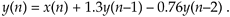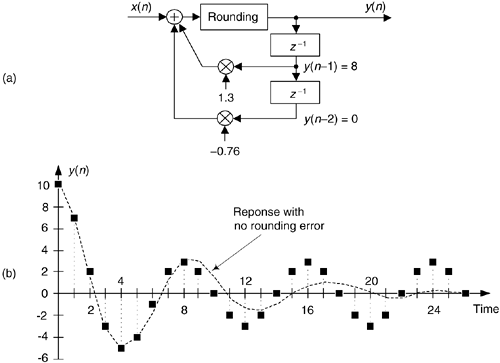# PITFALLS IN BUILDING IIR FILTERS

There's an old saying in engineering: "It's one thing to design a system on paper, and another thing to actually build one and make it work." (Recall the Tacoma Narrows Bridge episode!) Fabricating a working system based on theoretical designs can be difficult in practice. Let's see why this is often true for IIR digital filters.

Again, the IIR filter structures in Figures 6-18, and 6-22 are called Direct Form implementations of an IIR filter. That's because they're all equivalent to directly implementing the general time domain expression for an Mth-order IIR filter given in Eq. (6-21). As it turns out, there can be stability problems and frequency response distortion errors when direct form implementations are used for high-order filters. Such problems arise because we're forced to represent the IIR filter coefficients and results of intermediate filter calculations with binary numbers having a finite number of bits. There are three majors categories of finite-wordlength errors that plague IIR filter implementations: coefficient quantization, overflow errors and roundoff errors.

Coefficient quantization (limited-precision coefficients) will result in filter pole and zero shifting on the z-plane, and a frequency magnitude response that may not meet our requirements. The response distortion worsens for higher-order IIR filters.

Overflow, the second finite-wordlength effect that troubles IIR filters, is what happens when the result of an arithmetic operation is too large to be represented in the fixed-length hardware registers assigned to contain that result. Because we perform so many additions when we implement IIR filters, overflow is always a potential problem. With no precautions being made to handle overflow, large nonlinearity errors can result in our filter output samples—often in the form of overflow oscillations.

The most common way of dealing with binary overflow errors is called roundoff, or rounding, where a data value is represented by, or rounded off to, the b-bit binary number that's nearest the unrounded data value. It's usually valid to treat roundoff errors as a random process, but conditions occur in IIR filters where rounding can cause the filter output to oscillate forever, even when the filter input sequence is all zeros. This situation, caused by the roundoff noise being highly correlated with the signal (going by the names limit cycles and deadband effects) has been well analyzed in the literature[26,27]. We can demonstrate limit cycles by considering the second-order IIR filter in Figure 6-36(a), whose time domain expression isFigure 6-36. Limit cycle oscillations due to rounding: (a) second-order IIR filter; (b) one possible time domain response of the IIR filter.Let's assume this filter rounds the adder's output to the nearest integer value. If the situation ever arises where y(–2) = 0, y(–1) = 8, and x(0) and all successive x(n) inputs are zero, the filter output goes into endless oscillation, as shown in Figure 6-36(b). If this filter were to be used in an audio application, when the input signal went silent the listener could end up hearing an audio tone instead of silence. The dashed line in Figure 6-36(b) shows the filter's stable response to this particular situation if no rounding is used. With rounding however, this IIR filter certainly lives up to its name.

There are several ways to reduce the ill effects of coefficient quantization errors and limit cycles. We can increase the word widths of the hardware registers that contain the results of intermediate calculations. Because roundoff limit cycles affect the least significant bits of an arithmetic result, larger word sizes diminish the impact of limit cycles should they occur. To avoid filter input sequences of all zeros, some practitioners add a dither sequence to the filter's input signal sequence. A dither sequence is a sequence of low amplitude pseudorandom numbers that interferes with an IIR filter's roundoff error generation tendency, allowing the filter output to reach zero should the input signal remain at zero. Dithering, however, decreases the effective signal to noise ratio of the filter output. Finally, to avoid limit cycle problems, we can just use an FIR filter. Because FIR filters by definition have finite-length impulse responses, and have no feedback paths, they cannot support output oscillations of any kind.

As for overflow errors, we can eliminate them if we increase the word width of hardware registers so overflow never takes place in the IIR filter. Filter input signals can be scaled (reduced in amplitude by multiplying signals within the filter by a factor less than one) so overflow is avoided, but this signal amplitude loss reduces signal to noise ratios. Overflow oscillations can be avoided by using saturation arithmetic logic where signal values aren't permitted to exceed a fixed limit when an overflow condition is detected[28,29]. It may be useful for the reader to keep in mind that when the signal data is represented in two's complement arithmetic, multiple summations resulting in intermediate overflow errors cause no problems if we can guarantee the final magnitude of the sum of the numbers is not too large for the final accumulator register. Of course, standard floating point and block floating point data formats can greatly reduce the errors associated with overflow oscillations and limit cycles. (We discuss floating point number formats in Section 12.4.)

These quantized coefficient and overflow errors, caused by finite-width words, have different effects depending on IIR filter structure used. Referring to Figure 6-22, practice has shown the Direct Form II structure to be the most error-prone implementation.

The most popular technique for minimizing the errors associated with finite-word widths is to design IIR filters comprising a cascade string, or parallel combination, of low-order filters. The next section tells us why.

URL http://proquest.safaribooksonline.com/0131089897/ch06lev1sec7Amazon